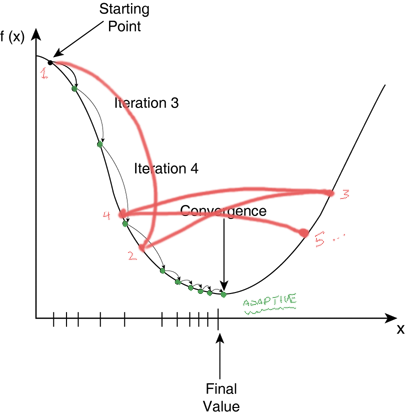# How can high learning rate diverge?

(Kyle Fang) #1

We have seems something similar to this, even in classThis is from DeepLearning-LecNotes2

Is this graph accurate?

I’m assuming we are talking about constant learning rate, but in these graphs, it likes like the learning rate is actually growing?

I’m more convinced withfrom here

But if the second graph is the truth, then high learning rate will not cause it to diverge right, it will just stabilize at a relatively higher error rate?

Or is there some math behind it, and graph one is the real deal?

0 Likes

(nok) #2

Imagine it is a basket, if you just slam the ball it will bounce off to elsewhere instead of bouncing in the basket.

1 Like

(William Horton) #3

It’s not that the learning rate is growing, it’s that the size of the step is equal to the learning rate multiplied by the gradient, and for this curve the gradient increases (curve gets steeper) as you get further from the minimum, so your steps (the red lines in the diagram) keep getting bigger.

3 Likes

#4

Also note that this is just a primitive (1-dimensional) sketch of what happens in deep learning. Instead of one parameter you are optimizing millions.

0 Likes

(Prash Goel) #5

I hope this helps:

0 Likes